Curve Drawing toolbar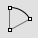Arc

Draw an arc with options for center, start, angle, and direction.

Link to Arc toolbarArc, Start, End, Direction

Arc by start, end, direction at start.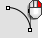Arc, Start, Direction, End

Arc by start, direction at start, end.Arc, Start, End, Point

Arc by start, end, point on arc.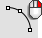Arc, Start, Point, End

Arc by start, point on arc, end.Arc, Tangent

Arc tangent to curves.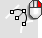Arc, Tangent, Tangent, Radius

Arc tangent, tangent, radius.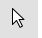Cancel

Cancel the current command and deselects objects.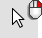Cancel, All

Turns off Points, CurvatureGraph, EMap, Zebra, MoveUVN, CurvatureAnalysis, and deselects objects.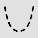Catenary

Creates a curve that a hanging chain or cable assumes under its own weight when supported only at its ends.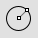Circle

Draw a circle from center and radius, diameter, points on the circumference, and circumference length.

Link to Circle toolbar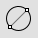Circle, Diameter

Draws a circle from points on diameter.Circle, Tangent, Tangent, Radius

Draws a circle tangent to two curves and a radius.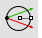Circle, Vertical to CPlane: center, radius

Draws a circle from a center and radius vertical to construction plane.Conic, Tangent at start

Draw a conic curve tangent at the start.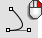Conic, Tangent at start and end

Draw a conic curve tangent at the start and end.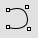Curve

Draw a curve from control point locations.

Link to Curve toolbar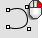CurveThroughPt

Fit a curve through point objects.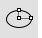Ellipse

Draw a closed elliptical curve from focus points, center and edges, bounding rectangle, and around a curve.

Link to Ellipse toolbar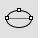Ellipse, Diameter

Draws a closed elliptical curve by diameter.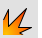Explode

Break objects down into components.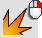ExtractSrf

Separate or duplicate a polysurface face.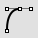HandleCurve

Draw chained Bézier curves with editing handles.Helix

Draw a helical curve with options for number of turns, pitch, vertical, reverse, and around a curve.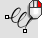Helix, Vertical

Draw a vertical helical curve.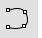InterpCrv

Fit a curve through picked locations.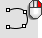HandleCurve

Draw chained Bézier curves with editing handles.InterpCrvOnSrf

Fit a curve through locations on a surface.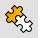Join

Connect curves, surface edges, or surfaces to form a single object.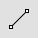Line

Draw a single line segment.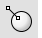Line, Normal

Draw a line segment normal to a surface.Line, Normal, BothSides

Draw a line segment normal to a surface from its midpoint.Line, Perpendicular, from curve

Draw a line segment perpendicular from a curve.Line, Perpendicular, to curve

Draw a line segment perpendicular to a curve.Line, Tangent

Draw a line segment tangent from a curve.Line, Vertical

Draw a line segment perpendicular to the construction plane.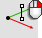Line, Vertical, BothSides

Draw a line segment perpendicular to the construction plane from its midpoint.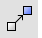Move

Move objects from one location to another.

Link to Transform toolbarParabola

Draw a parabolic curve from focus and vertex or endpoint.Parabola3Pt

Draw a parabolic curve through three picked points.Polygon

Draw a polygon with a specified number of sides with options for inscribed/circumscribed, by edge, star-shaped, around a curve, and vertical.

Link to Polygon toolbar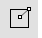Polygon, Square from center and corner

Draw a square polygon by center and corner.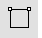Polygon, Square, from edge

Draw a square polygon by edge.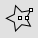Polygon, Star

Draw a polygon star.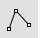Polyline

Draw a multi-segment polyline with options for line and arc segments, tracking line helpers, and close.

Link to Lines toolbar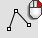Lines

Draw multiple adjoining line segments.Rectangle

Draw a rectangular closed polyline from with options for starting at the center, three points, vertical, and rounded with arc or conic corners.

Link to Rectangle toolbar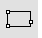Rectangle, 3Point

Draw a rectangle through three points.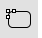Rectangle, Rounded

Draw a rectangle with rounded corners.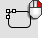Rectangle, Rounded, conic corners

Draw a rectangle with conic rounded corners.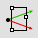Rectangle, Vertical

Draw a rectangle perpendicular to the construction plane.Sketch

Drag the mouse to draw a curve.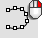Sketch, on surface

Drag the mouse to draw a curve on a selected surface.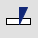Split

Divide objects using other objects as cutters.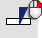Split, Isocurve

Divide objects using isocurves as cutters.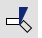Trim

Cut and delete selected portions of an object at the intersection with another object.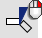Untrim

Remove trim curves from trimmed surfaces.

Rhinoceros 6 © 2010-2019 Robert McNeel & Associates. 05-Oct-2019